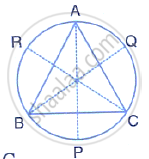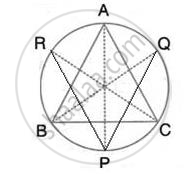Share

A Triangle Abc is Inscribed in a Circle. the Bisectors of Angles Bac, Abc and Acb Meet the Circumcircle of the Triangle at Points P, Q and R Respectively. Prove that :(I) ∠Abc = 2∠Apq, (Ii) ∠Acb = 2∠ - ICSE Class 10 - Mathematics

ConceptArc and Chord Properties - Angles in the Same Segment of a Circle Are Equal (Without Proof)

Question

A triangle ABC is inscribed in a circle. The bisectors of angles BAC, ABC and ACB meet the circumcircle of the triangle at points P, Q and R respectively. Prove that :
(i) ∠ABC = 2∠APQ,
(ii) ∠ACB = 2∠APR,
(iii) ∠QPR = 90 -1/2 ∠BACSolutionJoin PQ and PR

(i) BQ is the bisector of ∠ABC
⇒ ∠ABQ = 1/2 ∠ABC
Also, ∠APQ = ∠ABQ
(Angle in the same segment)
∴ ∠ABC = 2∠APQ

(ii) CR is the bisector of ACB
⇒ ∠ACR = 1/2 ∠ACB
Also, ∠ACR = ∠APR
(Angle in the same segment)
∴  ∠ACB = 2∠APR

(iii) Adding (i) and (ii)
We get
∠ABC + ∠ACB = 2 (∠APR + ∠APQ) = 2 ∠QPR

⇒ 180- ∠BAC = 2∠QPR
⇒∠QPR = 90 - 1/2 ∠BAC

Is there an error in this question or solution?
Solution A Triangle Abc is Inscribed in a Circle. the Bisectors of Angles Bac, Abc and Acb Meet the Circumcircle of the Triangle at Points P, Q and R Respectively. Prove that :(I) ∠Abc = 2∠Apq, (Ii) ∠Acb = 2∠ Concept: Arc and Chord Properties - Angles in the Same Segment of a Circle Are Equal (Without Proof).
S